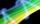# Coordinates of vector

Determine the coordinate of a vector u=CD if C(19;-7) and D(-16;-5)

Result

x =  -35
y =  2

#### Solution:Leave us a comment of example and its solution (i.e. if it is still somewhat unclear...):

Showing 0 comments:Be the first to comment!#### To solve this verbal math problem are needed these knowledge from mathematics:

For Basic calculations in analytic geometry is helpful line slope calculator. From coordinates of two points in the plane it calculate slope, normal and parametric line equation(s), slope, directional angle, direction vector, the length of segment, intersections the coordinate axes etc. Two vectors given by its magnitudes and by included angle can be added by our vector sum calculator.

## Next similar examples:

1. VectorDetermine coordinates of the vector u=CD if C[19;-7], D[-16,-5].
2. Vector - basic operationsThere are given points A [-9; -2] B [2; 16] C [16; -2] and D [12; 18] a. Determine the coordinates of the vectors u=AB v=CD s=DB b. Calculate the sum of the vectors u + v c. Calculate difference of vectors u-v d. Determine the coordinates of the vector w.
3. VectorsFor vector w is true: w = 2u-5v. Determine coordinates of vector w if u=(3, -1), v=(12, -10)
4. LineLine p passing through A[-10, 6] and has direction vector v=(3, 2). Is point B[7, 30] on the line p?
5. LineIt is true that the lines that do not intersect are parallel?
6. TeamsHow many ways can divide 16 players into two teams of 8 member?
7. SequenceWrite the first 6 members of these sequence: a1 = 5 a2 = 7 an+2 = an+1 +2 an
8. AverageIf the average(arithmetic mean) of three numbers x,y,z is 50. What is the average of there numbers (3x +10), (3y +10), (3z+10) ?
9. Three unknownsSolve the system of linear equations with three unknowns: A + B + C = 14 B - A - C = 4 2A - B + C = 0
10. Reference angleFind the reference angle of each angle:
11. Today in schoolThere are 9 girls and 11 boys in the class today. What is the probability that Suzan will go to the board today?
12. SequenceWrite the first 7 members of an arithmetic sequence: a1=-3, d=6.
13. Sequence 2Write the first 5 members of an arithmetic sequence a11=-14, d=-1
14. Elimination methodSolve system of linear equations by elimination method: 5/2x + 3/5y= 4/15 1/2x + 2/5y= 2/15
15. BlocksThere are 9 interactive basic building blocks of an organization. How many two-blocks combinations are there?
16. Three workshopsThere are 2743 people working in three workshops. In the second workshop works 140 people more than in the first and in third works 4.2 times more than the second one. How many people work in each workshop?
17. Theorem proveWe want to prove the sentence: If the natural number n is divisible by six, then n is divisible by three. From what assumption we started?